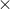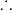In: Chemistry

# how manu mL of 5.00 M NaOH solution are needed to prepare 750mL of a 0.150...

how manu mL of 5.00 M NaOH solution are needed to prepare 750mL of a 0.150 M NaOH solution? and how many mL of water misy be added to prepare the above solution?

## Solutions

##### Expert Solution

We have to prepare diluted solution of NaOH ( 0.150 M ) from stock solution of NaOH ( 5.00 M ) . We can calculate volume of stock solution required to prepare dilute solution of NaOH by using dilution formula.

We have dilution formula, C stockV stock = C diluteV diluteV stock = C diluteV dilute / C stockV stock = 0.150 M750 ml / 5.00 M

V stock = 22.5 ml

To get 0.150 M NaOH solution , dilute 22.5 ml of 5.00 M NaOH to 750 ml by using distilled water.

Volume of water required to prepare 0.150 M NaOH solution = 750 ml - 22.5 = 727.5 ml

ANSWER : 727.5 ml water is required to add to 22.5 ml of 5.00 M NaOH solution to get 750 ml of 0.150 M NaOH solution.

## Related Solutions

##### A 25.0 mL sample of 0.150 M hydrofluoric acid is titrated with a 0.150 M NaOH...
A 25.0 mL sample of 0.150 M hydrofluoric acid is titrated with a 0.150 M NaOH solution. What is the pH at the equivalence point? The Ka of hydrofluoric acid is 6.8 × 10-4.
##### A 25.0-mL sample of 0.150 M hydrocyanic acid, HCN, is titrated with a 0.150 M NaOH...
A 25.0-mL sample of 0.150 M hydrocyanic acid, HCN, is titrated with a 0.150 M NaOH solution. What is the pH after 16.3 mL of base is added? The K a of hydrocyanic acid is 4.9 × 10 -10. A 25.0 mL sample of 0.150 M hydrocyanic acid, HCN, is titrated with a 0.150 M NaOH solution. What is the pH at the equivalence point? The K a of hydrocyanic acid is 4.9 × 10 -10. What is the pH...
##### How would you prepare 1.56L of a 0.120 M NaOH solution from a 1.20 M NaOH        solution?...
How would you prepare 1.56L of a 0.120 M NaOH solution from a 1.20 M NaOH        solution? A flask contains 55.0 mL of a hydrochloric acid solution. The molarity of the solution is not    known. It takes 23.5 mL of a 0.103 M sodium hydroxide solution to complete the reaction with hydrochloric acid. What is the molarity of the hydrochloric acid solution?
##### You prepare a diluted NaOH solution by adding 15 mL of 3 M NaOH to 435...
You prepare a diluted NaOH solution by adding 15 mL of 3 M NaOH to 435 mL of distilled water and stir for several minutes. In a clean dry beaker you obtain about 75 mL of standardized HCl and record the molarity. You take two burets, rinse one with the standardized HCl and one with the diluted NaOH solution. Fill one buret with the HCl and the other with NaOH. Prior to the titration the standard HCl soltion (~15.00 mL)...
##### Describe how to prepare 50 mL of solution buffered at pH 5.00. The buffer must be...
Describe how to prepare 50 mL of solution buffered at pH 5.00. The buffer must be designed so the pH changes no more than 0.10 units with the addition of 0.001 moles of acid or base. Choose from the following to design the buffer. Be sure to choose the buffer pair best suited to the assigned conditions. 1.acetic acid/sodium acetate 2.formic acid/sodium formate 3. benzoic acid/sodium benzoate please show your work-thank you!
##### A solution of HF is titrated with 0.150 M NaOH. The pH at the equivalence point...
A solution of HF is titrated with 0.150 M NaOH. The pH at the equivalence point will be greater than 7.0 less than 7.0 equal to 7.0 cannot be estimated from the information given.
##### Weigh by difference the amount needed to prepare 250 mL of a 0.01 M KIO3 solution...
Weigh by difference the amount needed to prepare 250 mL of a 0.01 M KIO3 solution and quantitatively transfer to a volumetric flask with the aid of a glass funnel. Record its mass to the nearest ±0.1 mg.
##### Consider the titration of of 5.00 mL of 0.450 M HBr using 0.120 M NaOH as...
Consider the titration of of 5.00 mL of 0.450 M HBr using 0.120 M NaOH as the titrant. a)Calculate the volume of 0.120 M NaOH that must be added in order to reach the equivalence point. b)Determine the pH of this titration solution at the equivalence point. c)Calculate the pH of this solution after 20.00 mL of 0.120 M NaOH has been added.
##### (4 pts) Determine the volume of 0.75 M stock solution needed to prepare 50.0 mL of...
(4 pts) Determine the volume of 0.75 M stock solution needed to prepare 50.0 mL of a 0.15 M solution. Show the complete calculation with units. *Note ‐ If you struggle with this calculation, refer to experiment 5. (8 pts) Write out a complete, detailed experimental procedure that you (or anyone else) could follow to carry out the solution preparation you have described above. Your procedure must follow these restrictions and guidelines:  You must prepare the 50.0 mL in...
##### How many 2 g tablets are needed to prepare 100 mL of a​ 10% solution?
How many 2 g tablets are needed to prepare 100 mL of a​ 10% solution?